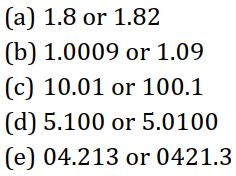# Ncert Class 6 Math Decimals Exercise 8.1

Decimals Class 6 Ex. 8.1

New Ncert Class 6, Chapter 8 Math Free Solution.

Exercise 8.1

Question 1 :- Which is greater?

(a) 0.3 or 0.4
(b) 0.07 or 0.02
(c) 3 or 0.8
(d) 0.5 or 0.05
(e) 1.23 or 1.2
(f) 0.099 or 0.19
(g) 1.5 or 1.50
(h) 1.431 or 1.490
(i) 3.3 or 3.300
(j) 5.64 or 5.603

Solution 1:-

(a) 0.3 or 0.4
0.3 < 0.4;

So, 0.4 is greater than 0.3

(b) 0.07 or 0.02
0.07 > 0.02;

So, 0.07 is greater than 0.02.

(c) 3 or 0.8
3 > 0.8;

So, 3 is greater than 0.8.

(d) 0.5 or 0.05
0.5 > 0.05

So, 0.5 is greater than 0.05.

(e) 1.23 or 1.2
1.23 > 1.2

So, 1.23 is greater than 1.2

(f) 0.099 or 0.19
0.099 < 0.19;

So, 0.19 is greater than 0.099.

(g) 1.5 or 1.50
1.5 = 1.50;

So,  1.5 is equals to 1.50.

(h) 1.431 or 1.490
1.431 < 1.490;

So, 1.490 is greater than 1.431.

(i) 3.3 or 3.300
3.3 = 3.300;
So, 3.3 is equals to 3.300.

(j) 5.64 or 5.603
5.64 > 5.603

So, 5.64 is greater than 5.603.

Question 2 :- Make five-example and find the greater number from them.Solution 2:-

(a) 1.8 or 1.82
1.8 < 1.82;

So, 1.82 is greater than 1.8.

(b) 1.0009 or 1.09
1.0009 < 1.09;

So, 1.09 is greater than 1.0009.

(c) 10.01 or 100.1
10.01 < 100.1;

So, 100.1 is greater than 10.01.

(d) 5.100 or 5.0100
5.100 > 5.0100;

So, 5.100 is greater than 5.0100.

(e) 04.213 or 0421.3
04.213 < 0421.3;

So, 0421.3 is greater than 04.213.